Home Practice
For learners and parents For teachers and schools
Textbooks
Full catalogue
Pricing SupportLog in

We think you are located in United States. Is this correct?

# 5.3 Properties of light: revision

## 5.3 Properties of light: revision (ESBMZ)

When light interacts with objects or a medium, such as glass or water, it displays certain properties: it can either be reflected, absorbed or transmitted.

### Reflection (ESBN2)

When you smile into a mirror, you see your own face smiling back at you. This is caused by the reflection of light rays on the mirror. Reflection occurs when an incoming light ray bounces off a surface. In the following figure, a still lake reflects the landscape surrounding it.

To describe the reflection of light, we will use the following terminology. The incoming light ray is called the incident ray. The light ray moving away from the surface is the reflected ray. The most important characteristic of these rays is their angles in relation to the reflecting surface. These angles are measured with respect to the normal of the surface. The normal is an imaginary line perpendicular to the surface. The angle of incidence, $$\theta_i$$ is measured between the incident ray and the surface normal. The angle of reflection, $$\theta_r$$ is measured between the reflected ray and the surface normal. This is shown in Figure 5.3.Figure 5.3: The angles of incidence and reflection are measured with respect to the normal to the surface.

When a ray of light is reflected, the reflected ray lies in the same plane as the incident ray and the normal. This plane is called the plane of incidence and is shown in Figure 5.4.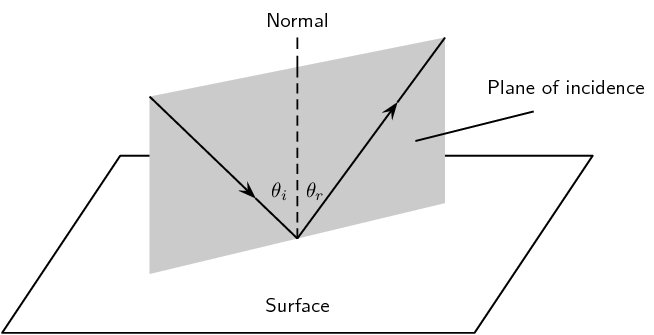Figure 5.4: The plane of incidence is the plane including the incident ray and the normal to the surface. The reflected ray also lies in the plane of incidence.
Law of reflection

The angle of incidence is equal to the angle of reflection:

$\theta_{i} = \theta_{r}$

and the incident ray, reflected ray, and the normal, all lie in the same plane.

The simplest example of the law of reflection is if the angle of incidence is $$\text{0}$$$$\text{°}$$. In this case, the angle of reflection is also $$\text{0}$$$$\text{°}$$. You see this when you look straight into a mirror.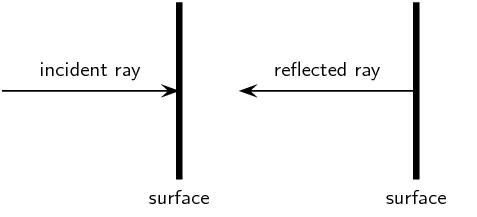Figure 5.5: When a light ray strikes a surface at right angles to the surface, then the ray is reflected directly back.

Applying what we know from the law of reflection, if a light ray strikes a surface at $$\text{60}$$$$\text{°}$$ to the normal to the surface, then the angle that the reflected ray makes with the normal must also be $$\text{60}$$$$\text{°}$$ as shown in Figure 5.6.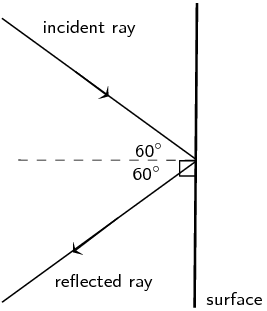Figure 5.6: Ray diagram showing angle of incidence and angle of reflection. The law of reflection states that when a light ray reflects off a surface, the angle of reflection $$\theta_r$$ is the same as the angle of incidence $$\theta_i$$.
temp text

#### Real world applications of reflection

A parabolic reflector is a mirror or dish (e.g. a satellite dish) which has a parabolic shape. Some examples of very useful parabolic reflectors are car headlamps, spotlights, telescopes and satellite dishes. In the case of car headlights or spotlights, the outgoing light produced by the bulb is reflected by a parabolic mirror behind the bulb so that it leaves as a collimated beam (i.e. all the reflected rays are parallel). The reverse situation is true for a telescope where the incoming light from distant objects arrives as parallel rays and is focused by the parabolic mirror to a point, called the focus, where an image can be made. The surface of this sort of reflector has to be shaped very carefully so that the rays all arrive at the same focal point.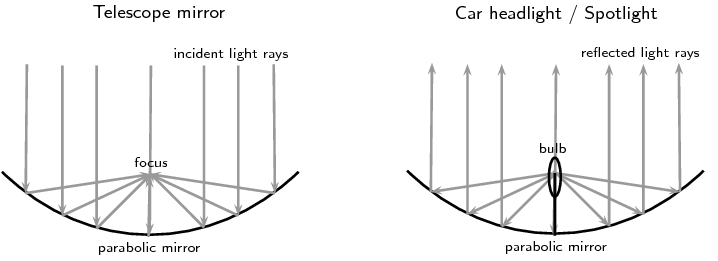Figure 5.7: On the left is a ray diagram showing how a telescope mirror works to collect incoming incident light (parallel rays) from a distant object such as a star or galaxy and focus the rays to a point where a detector e.g. a camera, can make an image. The diagram on the right shows how the same kind of parabolic reflector can cause light coming from a car headlight or spotlight bulb to be collimated. In this case the reflected rays are parallel.

## Rays and Reflection

Textbook Exercise 5.1

Are light rays real? Explain.

Light rays are not real.

In physics we use the idea of a light ray to indicate the direction in which light travels. In geometrical optics, we represent light rays with straight arrows to show how light propagates. Light rays are not an exact description of the light; rather they show the direction in which the light wavefronts are travelling.

The diagram shows a curved surface. Draw normals to the surface at the marked points.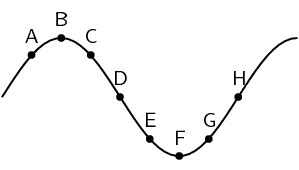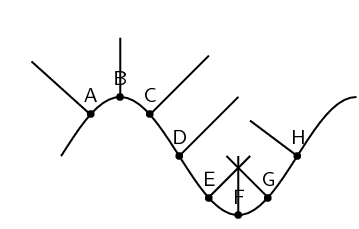Which of the labels, A-H, in the diagram, correspond to the following: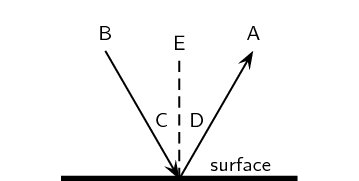normal
E
angle of incidence
C
angle of reflection
D
incident ray
B
reflected ray
A

State the Law of Reflection. Draw a diagram, label the appropriate angles and write a mathematical expression for the Law of Reflection.

The law of reflection states that the angle of incidence is equal to the angle of reflection and the incident ray, reflected ray, and the normal, all lie in the same plane.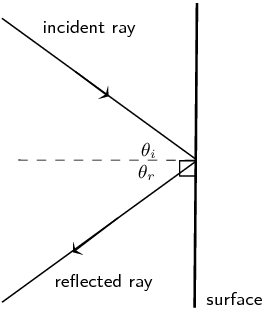We can write this mathematically as: $$θ_{i} = θ_{r}$$.

Draw a ray diagram to show the relationship between the angle of incidence and the angle of reflection.The diagram shows an incident ray $$I$$. Which of the other 5 rays (A, B, C, D, E) best represents the reflected ray of $$I$$?Ray B looks like it is reflected at the same angle as the incident ray.

A ray of light strikes a surface at $$\text{15}$$$$\text{°}$$ to the normal to the surface. Draw a ray diagram showing the incident ray, reflected ray and surface normal. Calculate the angles of incidence and reflection and fill them in on your diagram.

We are told that the ray of light strikes the surface at $$\text{15}$$$$\text{°}$$ to the normal to the surface, so the angle of incidence is $$\text{15}$$$$\text{°}$$. Since the angle of incidence is equal to the angle of reflection, the angle of reflection is also $$\text{15}$$$$\text{°}$$.

Putting this onto a ray diagram (not to scale!):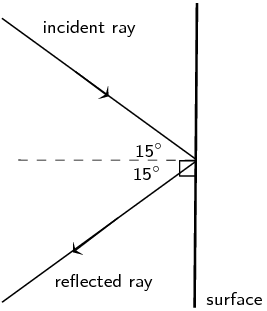A ray of light leaves a surface at $$\text{45}$$$$\text{°}$$ to the normal to the surface. Draw a ray diagram showing the incident ray, reflected ray and surface normal. Calculate the angles of incidence and reflection and fill them in on your diagram.

We are told that the ray of light leaves the surface at $$\text{45}$$$$\text{°}$$ to the normal to the surface, so the angle of reflection is $$\text{45}$$$$\text{°}$$. Since the angle of reflection is equal to the angle of incidence, the angle of incidence is also $$\text{15}$$$$\text{°}$$.

Putting this onto a ray diagram (not to scale!):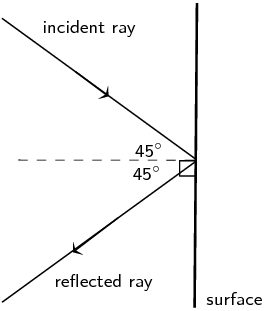A ray of light strikes a surface at $$\text{25}$$$$\text{°}$$ to the surface. Draw a ray diagram showing the incident ray, reflected ray and surface normal. Calculate the angles of incidence and reflection and fill them in on your diagram.

We are told that the ray of light strikes the surface at $$\text{25}$$$$\text{°}$$ to the surface. To find the angle of incidence we note that the normal is drawn at $$\text{90}$$$$\text{°}$$ to the surface. So the angle of incidence is $$\text{90}\text{°} - \text{25}\text{°} = \text{65}\text{°}$$. Since the angle of incidence is equal to the angle of reflection, the angle of reflection is also $$\text{65}$$$$\text{°}$$.

Putting this onto a ray diagram (not to scale!):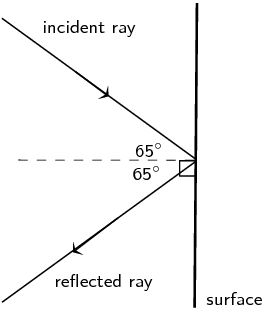A ray of light leaves a surface at $$\text{65}$$$$\text{°}$$ to the surface. Draw a ray diagram showing the incident ray, reflected ray and surface normal. Calculate the angles of incidence and reflection and fill them in on your diagram.

We are told that the ray of light leaves the surface at $$\text{65}$$$$\text{°}$$ to the surface. To find the angle of reflection we note that the normal is drawn at $$\text{90}$$$$\text{°}$$ to the surface. So the angle of reflection is $$\text{90}\text{°} - \text{65}\text{°} = \text{25}\text{°}$$. Since the angle of reflection is equal to the angle of incidence, the angle of incidence is also $$\text{25}$$$$\text{°}$$.

Putting this onto a ray diagram (not to scale!):A beam of light (for example from a torch) is generally not visible at night, as it travels through air. Try this for yourself. However, if you shine the torch through dust, the beam is visible. Explain why this happens.

To see the beam from a torch the light rays emitted by the torch need to be reflected off something for our eye to see it. At night the light rays are not being reflected off anything and so we do not see the beam of the torch. If we shine the torch through dust, then we can see the beam as the light rays reflect off the dust particles.

If a torch beam is shone across a classroom, only students in the direct line of the beam would be able to see that the torch is shining. However, if the beam strikes a wall, the entire class will be able to see the spot made by the beam on the wall. Explain why this happens.

To see the beam from a torch the light rays emitted by the torch need to be reflected off something for our eye to see it. So only the students in the direct path of the light will see that the torch is shining. However as soon as the torch beam is reflected off the wall, the learners are all able to see the spot where the beam reflects.

A scientist looking into a flat mirror hung perpendicular to the floor cannot see her feet but she can see the hem of her lab coat. Draw a ray diagram to help explain the answers to the following questions:

Will she be able to see her feet if she backs away from the mirror?

She will not be able to see her feet. Your eye sights along a line to see your feet. This line must intersect the mirror.

The solid lines show the path of light from her labcoat hem to her eyes, while the dotted line shows the path that light would have to take to reach her eyes from her feet. As she moves further away the only thing that changes is the angles of incidence and reflection.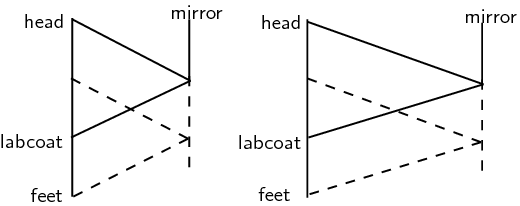What if she moves towards the mirror?

She still will not be able to see her feet. Your eye sights along a line to see your feet. This line must intersect the mirror.

The solid lines show the path of light from her labcoat hem to her eyes, while the dotted line shows the path that light would have to take to reach her eyes from her feet. As she moves closer the only thing that changes is the angles of incidence and reflection.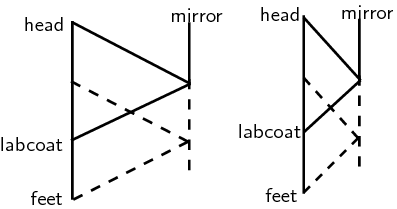#### Absorption

In addition to being reflected, light can also be absorbed. Recall from grade 10 that visible light covers a range of wavelengths in the electromagnetic spectrum. The colours we see with our eyes correspond to light waves with different wavelengths or frequencies.

Imagine that you shine a torch or other source of white light onto a white piece of paper. The light is reflected off the paper and into our eyes and we see the colour of the paper as white. Now if we were to shine the same light onto a red apple we will notice that the colour of the apple appears red. This means that the surface of the apple is only reflecting red light into our eyes. All the other wavelengths in the incident white light are absorbed by the apple's skin. If you touch the apple, it will feel warm because it is absorbing the energy from all the light it is absorbing. Because of absorption and reflection, we can perceive colours of different objects. White objects reflect all or most of the wavelengths of light falling on them, coloured objects reflect particular wavelengths of light and absorb the rest. Black objects absorb all the light falling on them. This is why wearing a white t-shirt outside in the sun is cooler than wearing a black t-shirt, since the white t-shirt reflects most of the light falling on it, while the black t-shirt will absorb it and heat up.

#### Transmission

A further property of light is that it can be transmitted through objects or a medium. Objects through which light can be transmitted are called transparent and objects which block out light or that light cannot pass through are called opaque.

For example, glass windows allow visible light to pass through them which is why we can see through windows. The light rays from things outside the window can pass through or be transmitted through the glass and into our eyes. Brick walls on the other hand are opaque to visible light. We cannot see through brick walls because the light cannot be transmitted through the wall into our eyes. The transmission of light through an object depends on the wavelength of the light. For example, short wavelength visible light cannot be transmitted through a brick wall whereas long wavelength radio waves can easily pass through walls and be received by a radio or cell phone. In other words, a brick wall is transparent to radio waves!